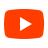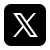## DAV PUBLIC SCHOOL, jamui

Affilliated by Prolific Educational Institutions of India.

MATHS

## Classes

### Lecture Series to all Senior Secondary Teachers  Chapter-1 (Relations and Functions), PPT-1, PPT-2, Worksheet-1, Worksheet-2, Worksheet-3,                    Worksheet-4, Worksheet-5  Chapter-2 (Inverse Trigonometric Functions), Worksheet  Chapter-3 (Matrix)-PPT, Video  Chapter-4 (Determinants)-PPT, Worksheet-1, Worksheet-2, Worksheet-3  Chapter-5 (Continuity and Differentiability-PPT, Worksheet (Basic), Worksheet (Standard),                     Worksheet (Advance)  Chapter-6 (Application of Derivatives), PPT, PPT-2 Worksheet (Basic-1, Basic-2),                     Worksheet (Standard-1, Standard-2), Worksheet (Advance-1)                    Application of Derivatives (Increasing and Decreasing)-PPT,                    Worksheet (Basic), Worksheet (Standard), Worksheet (Hots), Video,                    Video on Approximation                          Application of Derivatives (Maxima Minima)-PPT,                    Worksheet (Basic), Worksheet (Standard), Worksheet (Hots), Video                    Application of Derivatives (Tangents and Normals-PPT),                    Worksheet (Basic), Worksheet (Standard), Worksheet (Advance)  Chapter-7 (Definite Integral)-PPT, Worksheet (Basic), Worksheet (Standard), Worksheet (Advance),                    Definite and Indefinite Integral-PPT, Worksheet (Basic), Worksheet (Standard),                    Worksheet (Hots), Book  Chapter-8 (Area under the curve)-PPT, Worksheet (Basic), Worksheet (Standard),                    Worksheet (Advance), Video  Chapter-9 (Differential Equations)-PPT, Worksheet (Basic), Worksheet (Standard),                    Worksheet (Advance), Video  Chapter-10 (Vector Algebra) Worksheet (Basic), Worksheet (Standard), Worksheet (Hots) Chapter-11 (Three Dimensional Geometry-PPT), Worksheet (Basic), Worksheet (Standard),                     Worksheet (Hots), Mind Map Chapter-12 (Linear Programming)

DAV PUBLIC SCHOOL, JAMUI